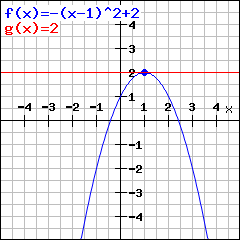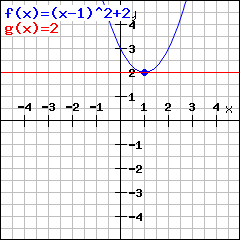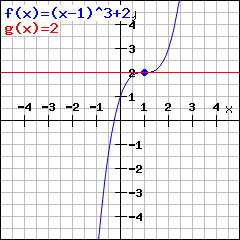# Change of signsEnter your function here. Hint: Enteras 3*x^2 ,as 3/5 andas (x+1)/(x-2x^4)

## What is the use of the change of sign?

By checking for the change of sign, you can check whether a function with derivativehas a maximum / minimum turning point or a saddle point. Here are three examples where the function has slopein (1|2):This function has slopein (1|2) and a maximum turning point. Atthe graph ascends, i.e. the derivative is larger thanin here. Atthe Graph falls, i.e. the derivative is less than. This means that around a maximum turning point, the sign of the derivative is + before and - after the turning point. This means the derivative changes signs from + to - .This function has slopein (1|2), but a minimum turning point. Atthe graph falls, i.e. the derivation here is less than. Atthe graph ascends, i.e. the derivation is larger than. This implies that at a minimum turning point, the sign of the derivation is - before and + after the turning point. This means the derivation changes signes from - to +.This function also has slopeat (1|2), but no turning point. You see that the graph ascends atas well as at. This implies you have no turning point if the derivation does not change signs. Such a point (that is no turning point but has derivation) is called saddle point.

## How to use the change of sign criterion?

The derivation beingis necessary for a turning point (i.e. it is always the case at a turning point). But it is not necessary, what means, just because the derivation is, there does not have to be a turning point (check out for the saddle point above).
If the derivation is not only, but also changes signs, then you have to have a turning point. In mathematics, we say that derivationand change of signs is sufficient for having a turning point.